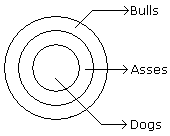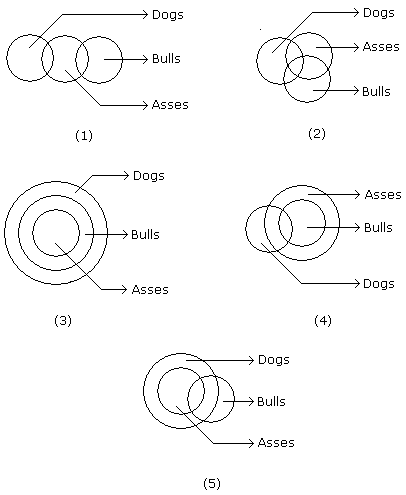# Verbal Reasoning - Syllogism

Exercise : Syllogism - Introduction
Directions to Solve

In each of the following questions two statements are given and these statements are followed by two conclusions numbered (1) and (2). You have to take the given two statements to be true even if they seem to be at variance from commonly known facts. Read the conclusions and then decide which of the given conclusions logically follows from the two given statements, disregarding commonly known facts.

• (A) If only (1) conclusion follows
• (B) If only (2) conclusion follows
• (C) If either (1) or (2) follows
• (D) If neither (1) nor (2) follows and
• (E) If both (1) and (2) follow.

Introduction:

The questions which are asked in this section contain two or more statements and these statements are followed by two or more conclusions. You have to find out which of the conclusions logically follow from the given statements. The statements have to be taken true even if they seem to be at variance from the commonly known facts.

For such questions, you can take the help of Venn Diagrams. On the basis of the given statements, you should draw all the possible diagrams, and then derive the solution from each of these diagrams separately. Finally, the answer common to the all the diagrams is taken.

Example 1:

Statements:

1. All dogs are asses.
2. All asses are bulls.

Conclusions:

1. Some dogs are not bulls.
2. Some bulls are dogs.
3. All bulls are dogs.
4. All dogs are bulls.

Solution:

On the basis of both statements, the following one diagram is possible.From the diagram it is clear that (2) and (4) conclusions logically follow.

Example 2:

Statements:

1. Some dogs are asses.
2. Some asses are bulls.

Conclusions:

1. Some asses are not dogs.
2. Some dogs are bulls.

Solution:

From these given statements the following diagrams are possible:From the diagram neither (1) nor (2) conclusions follow.